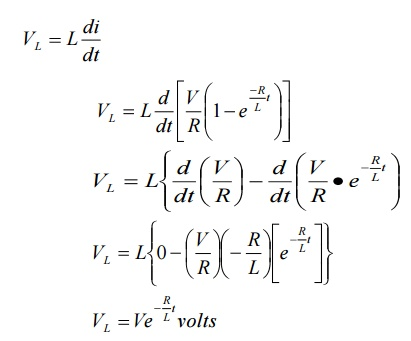Home | | Circuit Theory | Time constant and step response of series RL circuit

# Time constant and step response of series RL circuit

What is time constant? Explain time constant in case of series RL circuit. Or A series RL circuit with initial current I0 in the inductor is connected to a dc voltage V at t = 0. Derive the expression for instantaneous current through the Inductor for t>0. Or Explain in brief about the step response of series RL circuits.

1) What is time constant? Explain time constant in case of series RL circuit.

Or

2) A series RL circuit with initial current I0 in the inductor is connected to a dc voltage V at t = 0. Derive the expression for instantaneous current through the Inductor for t>0.

Or

3) Explain in brief about the step response of series RL circuits.

The response or the output of the series RL and RC circuits driven dc excitations is called step response of the network.

Consider that a dc voltage is applied to any general network through a switch k as shown

in fig.Initially switch k is kept open for very long time. So no voltage is applied to the network. Thus the voltage at input-terminals of network is zero. So we can write voltage across terminals A and B V

(l) is zero. When the switch k is closed at t=0, the dc voltage v gets applied to the network. The voltage across terminals A and B suddenly or instantaneously rises to voltage V. the variation of voltage across terminals +1 and B against time t as shown in fig (b).

In fig (b) it is observed that at t=0, there is a step of V volts. Such signal or function is called step function. We can define step function asWhen the magnitude of the voltage applied is 1 volt then the function is called unit step function.

When the circuits are driven by driving sources, then such circuits are called driven circuits. When the circuits are without such driving sources, then such circuits are called undriven circuits or

source free circuits.

Step response of Driver series RL circuit:-

Consider a series RL circuit.

At t=0-, switch k is about to close but not fully closed. As voltage is not applied to the circuit, current in the circuit will be zero.In this current through inductor can not change instantaneously.Let initial current through inductor can be represented as I0. in above case I0 is zero. Assume that switch k is closed at t =0.From above fig (a) shows variation of current I with respect to time (t) i.e. current increases exponentially with respect to time. The rising current produces rising flux, which induces emf in coil. According to Lens’s law, the self induced emf opposes the flow of current. Because of this induced emf and its opposition, the current in the coil don’t reach its max value.

The point p shown on graph indicates that current in circuit rises to 0.632 time’s maximum value of current in steady state.

“the time required for the current to rise to the 0.632 of its final value is known as time constant of given RL circuit. The time constant is denoted by z”. Thus for series RL circuit, time constant isThe initial rate of rise of current is large up to first time constant. At later stage, the rate of rise of current reduces.

Theoretically I reach maximum value after infinite time.

Voltage across inductor L is given byStudy Material, Lecturing Notes, Assignment, Reference, Wiki description explanation, brief detail
Electrical and electronics : Circuit Theory : Transient Response For DC Circuits : Time constant and step response of series RL circuit |